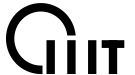﻿ 单面SMT贴装工艺及2D双面SMT贴片流程介绍 - 全球威

#PCBA高端定制供应商SMT贴片加工、DIP插件后焊、OEM、EMS加工制造一条龙优质服务

1881877101013143439010

## 单面SMT贴装工艺及2D双面SMT贴片流程介绍

内容来自:www.pcba-smt.cn

A、进货检验=>屏幕焊锡膏印刷在PCB A面（分配表面贴装胶）=>丝网焊膏印刷在PCB B面（分配表面贴装胶） =>表面贴装=>干燥（凝固）=>回流焊接（B侧更好）=>清理=> VI＆amp; AOI =>返工/修复。 

< strong> B。进货检验=> PCB A侧的屏幕焊膏印刷（分配表面贴装胶）=>表面贴装=>干燥（凝固）=> A侧回流焊=>清洁=>板周转=>在PCB B侧分配表面贴装粘合剂=>表面贴装=>凝固=> B侧波浪焊接=>清理=> VI＆amp; AOI =>返工/修复。

https://www.pcba-smt.cn/

https://www.pcba-smt.cn/

<强>3单面混合工艺：

https://www.pcba-smt.cn/

<强>4。 D双面混合流程： 

A、进货检验=>在PCB B侧分配表面贴装粘合剂=>表面贴装=>凝固=>板材周转=> PCBA侧的DIP =>波峰焊接=>清理=> VI＆amp; AOI =>返工/修复。

https://www.pcba-smt.cn/

< strong> B。进货检验=> PCBA侧的DIP（弯曲销）=>板材周转=>在PCB B侧分配表面贴装粘合剂=>表面贴装=>凝固=>板材周转=>波峰焊接=>清理=> VI＆amp; AOI =>返工/修复。

C、进货检验=> PCB A侧的屏幕焊膏印刷=>表面贴装=>干燥=> ReflowSoldering => DIP（弯曲销）=>板材周转=>分配表面贴装粘合剂PCB B侧=>表面安装=>凝固=>板材周转=>波峰焊接=>清理=> VI＆amp; AOI =>返工/修复A侧混合，B侧安装。

D、进货检验=>屏幕焊锡膏在PCB B侧印刷=>表面贴装=>凝固=>印版翻转=>屏幕焊锡膏印刷在PCB A侧=>表面贴装=> PCB A侧的回流焊接=> DIP => B侧的波峰焊接=>清除=> VI＆amp; AOI =>返工/修复A侧混合，B侧安装。



E、进货检验=>屏幕焊锡膏印刷在PCB上B侧（分配表面贴装粘合剂）=>表面贴装=>干燥（凝固）=>回流焊接=>印版转换=>丝网焊膏印刷在PCB A侧=>表面贴装=>干燥=>回流焊接1（可能是部分焊接）=> DIP =>波峰焊2（如果组件很少，可以使用手工焊接）=>清理=> VI＆amp; AOI =>返工/修理侧面安装，B侧混合。



5、双面PCB组装工艺

A、进货检验=> PCB A侧的屏幕焊膏印刷（分配表面贴装粘合剂=>表面贴装=>干燥（凝固）=>回流焊接A侧=>清洁=>印版翻转=>屏幕焊膏印刷在PCB B上侧面（分配表面贴装粘合剂）=>表面贴装=>干燥=>回流焊接（B侧更好）=>清理=> VI＆amp; AOI =>返工/修复。

B、进货检验=> PCB A侧的屏幕焊膏印刷（分配表面贴装粘合剂=>表面贴装=>干燥（凝固）=>回流焊接面A侧=>清洁=>板材周转=>屏幕PCB B侧的焊膏印刷（分配表面贴装粘合剂）=>表面贴装=>凝固=> B侧波峰焊接=>清理=> VI＆amp; AOI =>返工/修复。

https://www.pcba-smt.cn/

## 新闻资讯news CENTER扫一扫，更多精彩